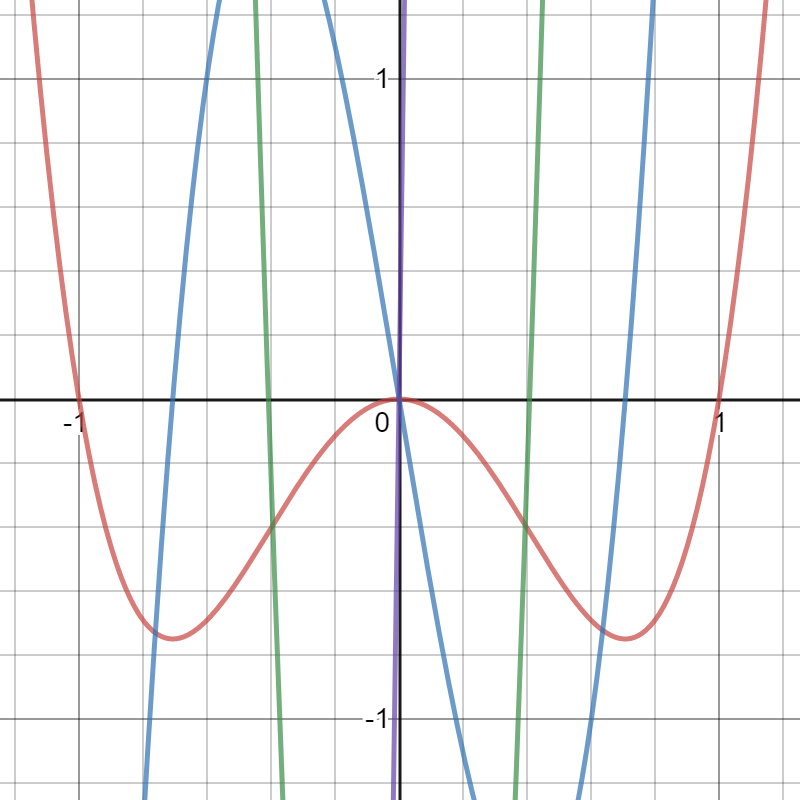# Fifth Derivative (Crackle)

The derivative of the fourth derivative f(4)(x) is called the fifth derivative. It is denoted as f(5)(x).

This is an uncommon derivative, but it’s sometimes used in physics where it’s defined as the fifth derivative of the position function with respect to time.

## Fifth Derivative Example

Example question: Find the fifth derivative of 3x4 – 3x2.

Solution: Take the derivative five times. :

1. f′(x) = 12x3 – 6x (first derivative)
2. f′′(x) = 36x2 – 6 (second derivative)
3. f′′(x) = 72x (third derivative)
4. f4(x) = 72 (fourth derivative)
5. f5(x) = 0 (fifth derivative)

For this example, the function has exponents, so I used the Power Rule for differentiation for the first two steps. When the function differentiated into a constant function, I used the derivative of a constant rule.Graph of 3x4 – 3x2(red), f′(x) = 12x3 – 6x (blue), f′′(x) = 36x2 – 6 (green), f′′(x) = 72x (purple). The fourth derivative, f4(x) = 72 and fifth derivative, zero, are not shown on the graph.

## Snap, Crackle, and Pop

The fourth, fifth, and sixth derivatives are sometimes called snap, crackle, and pop respectively (in homage to Rice Krispies cereal). The terms were first mentioned by Codner et al, as cited in Scott, 1997 in a footnote:

“17. These terms were suggested by J. Codner, E. Francis, T. Bartels, J. Glass, and W. Jefferys, respectively, in response to a question posed on the
USENET sci.physics newsgroup.”

## References

Scott, J. Some Simple Chaotic Jerk Functions in Am. J. Phys., Vol. 65, No. 6, June.
Viljoen, C & Van der Merwe, L. (1999). Elementary Statistics: Calculations & Interest for Business & Economics. Pearson South Africa.

CITE THIS AS:
Stephanie Glen. "Fifth Derivative (Crackle)" From StatisticsHowTo.com: Elementary Statistics for the rest of us! https://www.statisticshowto.com/fifth-derivative-crackle/